# Simulating Stock Prices: A Naive Approach

Disclaimer: This project/post is for fun/education please don’t use the results of this project to make investment decisions. If you chose to ignore this disclaimer and do just that I am not responsible for the (very probable) large losses that may occur.

Simulating future stock prices and price movements has obvious uses in financial applications. One common way of simulating prices is to use Monte Carlo simulations and sampling random movements from a geometric Brownian motion process parameterized with data from historical stock data. I plan to explore this more in a future post. However, while building up the simulation for that post I decided to take a more naive approach to generate future time series values for the stock prices. In this post, I will talk about the approach I used and build up the Python implementation of the simulation.

## Gathering Data

In a previous post (also on Medium), I developed an API that takes advantage of Yahoo Finance’s ability to download historic stock data (closing price, open price, volume, etc.) via their website. I used that API in this project to retrieve the data for the stock prices. The implementation is given below and details are available via the links above.

import datetime
import time
import pandas as pd

class YahooAPI(object):
def __init__(self, interval="1d"):
self.interval = interval

def __build_url(self, ticker, start_date, end_date):
return self.base_url.format(ticker=ticker, start_time=start_date, end_time=end_date, interval=self.interval)

def get_ticker_data(self, ticker, start_date, end_date):
# must pass datetime into this function
epoch_start = int(time.mktime(start_date.timetuple()))
epoch_end = int(time.mktime(end_date.timetuple()))



## The Stock Class

To be able to easily work with the data from Yahoo Finance I created a custom class in Python: the Stock class. The entire purpose of this class is to store the data from Yahoo and calculate some statistics which will be used to parameterize Gaussian distributions from which we will draw a few random numbers.

The class constructor takes 2 parameters: the stock’s ticker symbol and the Yahoo Finance data (in Pandas dataframe format).

def __init__(self, symbol, data):
self.symbol = symbol
self.data = data
self.data.Date = pd.to_datetime(self.data.Date, format="%Y-%m-%d")
self.compute_stats()

This constructor is pretty straightforward, most of the computation is saved for the compute_stats() method called from the constructor. All that’s done here is storing the data and ensuring the Date column in our dataframe is a datetime object.

The compute_stats() function takes a single parameter which tells the method which header to look for when computing the statistics. The default is ‘Open’ so the program, by default, assumes the dataframe has an Open column.

def compute_stats(self, price_header='Open'):
self.ups = self.open_diffs[self.open_diffs > 0]
self.downs = self.open_diffs[self.open_diffs < 0]
self.none = self.open_diffs[self.open_diffs == 0]

self.count = len(self.data)
self.up_count = len(self.ups)
self.down_count = len(self.downs)
self.no_change_count = len(self.none)

self.avg_up = self.ups.mean()
self.avg_down = self.downs.mean()

self.var_up = self.ups.var()
self.var_down = self.downs.var()

As seen above, this class computes and stores a number of statistics including the variance and mean for the opening price and the upward and downward price movements. The price movements are determined by the built-in diff() method in Pandas. This method calculates the difference between two consecutive rows in a dataframe column. The differences are then sorted into up movements (positive difference), down movements (negative difference), and no change (no difference). This data will be used, as previously mentioned, to parameterize normal distributions later in the project. Mean and variance are defined as:

Mean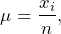Variance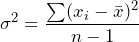where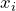is the stock price at timeand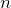is the number of stock prices/days under consideration.

Next we define a few functions that are used to help determine the path taken in the different Monte Carlo simulations.

def down_likelihood(self):
return float(self.down_count) / float(self.count)

def up_likelihood(self):
return float(self.up_count) / float(self.count)

def no_change_likelihood(self):
return float(self.no_change_count) / float(self.count)

def get_normal_dist_params(self):
"""
Returns mean and variance of ups and downs to parameterize a normal
distribution.
"""
params = {}
params['up'] = [self.avg_up, self.var_up]
params['down'] = [self.avg_down, self.var_down]
return params

The first three functions here are the likelihood of the stock price moving down, up, and not at all, respectively. These are defined as the number of times the stock made such a move divided by the total number of movements under consideration. The last method provides the parameters needed for a Gaussian distribution,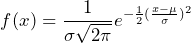where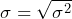is the standard deviation and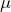is the average or arithmetic mean. These parameters are used in two separate distributions, one that models the average price movement upwards and one that models the average price movement downwards. Random samples are drawn from these distributions at each time step of the simulation to create a random path.

The full code for the Stock class can be found at the end of this post.

## Monte Carlo Simulations

Monte Carlo simulation is a way to model a stochastic process that cannot easily be predicted due to underlying sources of randomness. Monte Carlo simulations are widely used in many fields including energy, engineering, insurance, oil and gas transportation, and, as in our case, finance. These simulations provide risk analysis by building many different models of the process with different random values drawn from a (or a set of) probability distributions. In the case of stock price predictions, we are essentially creating many different paths the stock can take as time progresses and determining what an average path might look like.

To begin, we have to include a few libraries and set some simulation parameters. To set the ticker symbols you’re interested in for your simulations you only need to change the values of the list on line 16, highlighted below. Symbols can be added or removed from this list, there’s nothing magical about having 5 ticker symbols in the list.

from yahoo_api import YahooAPI
from stock import Stock

import datetime
import matplotlib.pyplot as plt
import pandas as pd
from numpy import random
import numpy as np
from dateutil.relativedelta import relativedelta

if __name__ == '__main__':
# parameters
days = 5        # number of days into the future to predict prices for
simulations = 50    # number of random paths to generate
# stock ticker symbols to fetch data for
symbols = ['fsly', 'icsh', 'aapl', 'intc', 'msft'] 

Next, we need to fetch the data from Yahoo Finance. As mentioned in my post about my Yahoo Finance API, the data is returned as a Pandas dataframe. After the data is fetched, a dictionary of predicted prices is created for each stock. The value of the dictionary’s key/value pair is a list of lists with the ‘child’ list storing a single element which is the most recent stock price. As the simulation progresses, each list is filled with predicted stock prices for each symbol/simulation run.

dh = YahooAPI(interval="1d")  # specify daily data from the api
portfolio = {}  # dictionary to store the stock data
current = datetime.datetime.today() # get current date
past = current - relativedelta(months=6)  # get 6 months worth of data
for sym in symbols:
df = dh.get_ticker_data(sym, past, current)
portfolio[sym] = Stock(sym, df)  # fetch and store the data

prices = {}
for sym in symbols:
prices[sym] = [[portfolio[sym].data.Open[len(portfolio[sym].data) -1]] \
for _ in range(simulations)]

Now that we have the data and have initialized an object to store the predicted trajectories, we can use the statistical data computed earlier to generate some potential stock price paths.

for sym in symbols:
stock = portfolio[sym]
# retrieve the parameters for the normal distributions
params = stock.get_normal_dist_params()
# get the list of lists to store the stock price trajectories
price_item = prices[sym]

for i in range(simulations):
## TODO: to improve this, use brownian motion for price change
for _ in range(days):
# determine if we're moving up, down, or not at all
up_down_neutral = random.uniform(0, 1)
if up_down_neutral < stock.down_likelihood():
# get the random downward price movement based on the
# statistical properties of past downward price movements
change = random.normal(loc=params['down'], scale=np.sqrt(params['down']))
if change > 0:
# we don't want to accidentally go up
change *= -1
# add the price movement to the list
price_item[i].append(price_item[i][len(price_item[i]) - 1] + change)
elif up_down_neutral >= stock.down_likelihood() and \
(up_down_neutral <= stock.up_likelihood() +  \
stock.down_likelihood()):
# get the random upward price movement based on the statistical
# properties of past upward price movements
change = random.normal(loc=params['up'], scale=np.sqrt(params['up']]))
if change < 0:
# we don't want to accidentally go down
change *= -1
# add the price movement to the list
price_item[i].append(price_item[i][len(price_item[i]) - 1] + change)
else:
# add the (lack of) price movement to the list
price_item[i].append(price_item[i][len(price_item[i]) - 1])

Now, in the prices dictionary, we should have(number of simulations) different trajectories for the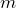different stock ticker symbols. Next, we need to calculate some valuable data from these different simulations then plot the results to determine where the method thinks the stock price is going to go next.

The first thing we’re going to do is determine the worst- and best-case scenarios presented by the Monte Carlo simulations. Again, we’re going to use dictionaries (they happen to be nifty in this context) where the keys are the stock ticker symbols and the values are indexes into the stock’s simulation list where the stock hits a maximum and minimum final value.

max_idx_dict = {}
min_idx_dict = {}
for sym in symbols:
max_amt = -1000000000   # small value
min_amt = 1000000000      # big value
last_idx = len(prices[sym]) - 1   # final stock value for simulations
for i in range(simulations):
price = prices[sym][i][last_idx]   # get final price
if price < min_amt: # lowest final price
min_amt = price
min_idx_dict[sym] = i
if price > max_amt: # highest final price
max_amt = price
max_idx_dict[sym] = i

Now it might be useful to know the average path predicted by the simulations. This will be taken as the sum over all simulations of the price predicted for each day divided by the number of simulations (again stored in a dictionary as described above).

avg_price_dict = {}
for sym in symbols:
# initialize with the last days' data (for plotting purposes)
avg_price_dict[sym] = [portfolio[sym].data.Open[len(portfolio[sym].data) - 1]]
for i in range(days):
avg = 0.0    # determine the average value for each day
for j in range(simulations):
avg += prices[sym][j][I]
# put the average price in the list of average prices (one for each
# predicted day)
avg_price_dict[sym].append(float(avg)/float(simulations))

With this information gathered and/or determined we’re ready to plot the results and see where we’ve predicted these stock prices to go.

## Results

For this project I’ve divided the results into three separate graphs: the simulations graph which shows each predicted path (sort of useless), the best-, worst- and average-case paths, and the distribution of the predicted prices broken up by day. The latter two graphs are probably the most useful and provide meaningful information about the predicted stock movements and their volatility.

Running 50 Monte Carlo simulations for the ticker symbols FSLY (Fastly), MSFT (Microsoft), ICSH (iCash), AAPL (Apple), and INTC (Intel) produces the following graphical output:

Of course, running the program locally allows you to play with the graph via utilities in Matplotlib which can make things a little easier to see. As mentioned above the first graph shows all of the predicted paths for each stock. This is a little useless since it’s hard to see what exactly is going on but it’s nice to include to help understand what the algorithm is doing.

The second graph shows the best-, worst-, and average-case trajectories for each stock. The worst-case trajectories are in red, the best-cases are green, and the average trajectory is orange. The data in the graph is essentially the entire point of running the simulations. We want to know, on average, given random price fluctuations, what we can expect the stock to do in the future. The validity of these graphs depends on how closely the random fluctuations match actual fluctuations in the market. However, this obviously doesn’t account for events that cause large swings in stock price (bad news, good news, earnings releases, etc.). To try to match the fluctuations, in this method, the average price change data from the past six months was used and it was assumed that similar fluctuations can be expected in the future. Then, normal distributions were parameterized with the mean and standard deviations of the upwards and downwards price movements. Drawing from these distributions provided the random movements for the predicted days.

The final graph shows how the predicted prices for each day were distributed across the simulations. This graph also provides valuable information in that it provides a look at how volatile the stock has been and is expected to be. A tight cluster of prices is indicative of a stock price that is not subject to wild mood swings since the probability distribution from which the data was drawn likely had a lower variance (standard deviation). This can also add confidence in the Monte Carlo predictions.

Below is another example run of the method. This time only the stock price for the company Fastly (FSLY) was simulated which makes the information in the graphs a little easier to see. Fastly has recently had a strong run-up in price so the graphs show the outcome of using a stock ticker symbol with large variations in price from day to day.

And for a third example, I choose the ticker symbol ICSH. ICSH is an ultra-short-term bond and cash fund that deviates very little in price but pays a reasonable dividend. This set of graphs shows an ETF with very little variation in the randomly selected prices. I know it doesn’t really look like it you but need to be mindful of the y-axis, for some reference I’ve also included the EFT with Prudential Financial (PRU), making it look like a straight line.

Information on how these graphs were created is given below in the Full Code section.

## Full Code

### YahooAPI

import datetime
import time
import pandas as pd

class YahooAPI(object):
def __init__(self, interval="1d"):
self.interval = interval

def __build_url(self, ticker, start_date, end_date):
return self.base_url.format(ticker=ticker, start_time=start_date, end_time=end_date, interval=self.interval)

def get_ticker_data(self, ticker, start_date, end_date):
# must pass datetime into this function
epoch_start = int(time.mktime(start_date.timetuple()))
epoch_end = int(time.mktime(end_date.timetuple()))

if __name__ == '__main__':
dh = YahooAPI()
now = datetime.datetime(2020, 6, 28)
then = datetime.datetime(2020, 1, 1)
df = dh.get_ticker_data("msft", then, now)
print(df)

### Stock Class

import pandas as pd

class Stock(object):
def __init__(self, symbol, data):
self.symbol = symbol
self.data = data
self.data.Date = pd.to_datetime(self.data.Date, format="%Y-%m-%d")
self.compute_stats()

def __validate_data(self):
# first row doesn't have a diff, so -1
if not ((self.up_count + self.down_count + self.no_change_count) == self.count-1):
raise Exception("Invalid statistics for", self.symbol + ".")

self.ups = self.open_diffs[self.open_diffs > 0]
self.downs = self.open_diffs[self.open_diffs < 0]
self.none = self.open_diffs[self.open_diffs == 0]

self.count = len(self.data)
self.up_count = len(self.ups)
self.down_count = len(self.downs)
self.no_change_count = len(self.none)

self.avg_up = self.ups.mean()
self.avg_down = self.downs.mean()

self.var_up = self.ups.var()
self.var_down = self.downs.var()

self.__validate_data()

def down_likelihood(self):
return float(self.down_count) / float(self.count)

def up_likelihood(self):
return float(self.up_count) / float(self.count)

def no_change_likelihood(self):
return float(self.no_change_count) / float(self.count)

def get_normal_dist_params(self):
"""
Returns mean and variance of ups and downs to parameterize a normal
distribution.
"""
params = {}
params['up'] = [self.avg_up, self.var_up]
params['down'] = [self.avg_down, self.var_down]
return params

### Simulation Logic

from yahoo_api import YahooAPI
from stock import Stock

import datetime
import matplotlib.pyplot as plt
import pandas as pd
from numpy import random
import numpy as np
from dateutil.relativedelta import relativedelta

if __name__ == '__main__':
# parameters
savings_rate = 0.0125
days = 5
simulations = 50
data_months = 1

dh = YahooAPI(interval="1d")
# some of the oldest s&p 500 (spy;1993), cash (shv;2007), and corporate (lqd;2002) bond etfs
symbols = ['icsh', 'pru'] #, 'icsh', 'aapl', 'intc', 'msft']
portfolio = {}

current = datetime.datetime.today()
past = current - relativedelta(months=6)
for sym in symbols:
df = dh.get_ticker_data(sym, past, current)
portfolio[sym] = Stock(sym, df)

prices = {}
for sym in symbols:
prices[sym] = [[portfolio[sym].data.Open[len(portfolio[sym].data) -1]] \
for _ in range(simulations)]

for sym in symbols:
stock = portfolio[sym]
params = stock.get_normal_dist_params()
price_item = prices[sym]

for i in range(simulations):
## TODO: to improve this, use brownian motion for price change
for _ in range(days):
up_down_neutral = random.uniform(0, 1)
if up_down_neutral < stock.down_likelihood():
change = random.normal(loc=params['down'], scale=np.sqrt(params['down']))
if change > 0:
change *= -1
price_item[i].append(price_item[i][len(price_item[i]) - 1] + change)
elif up_down_neutral >= stock.down_likelihood() and \
(up_down_neutral <= stock.up_likelihood() + \
stock.down_likelihood()):
change = random.normal(loc=params['up'], scale=np.sqrt(params['up']))
if change < 0:
change *= -1
price_item[i].append(price_item[i][len(price_item[i]) - 1] + change)
else:
price_item[i].append(price_item[i][len(price_item[i]) - 1])

max_idx_dict = {}
min_idx_dict = {}
for sym in symbols:
max_amt = -1000000000
min_amt = 1000000000
last_idx = len(prices[sym]) - 1
for i in range(simulations):
price = prices[sym][i][last_idx]
if price < min_amt: # lowest final price
min_amt = price
min_idx_dict[sym] = i
if price > max_amt: # highest final price
max_amt = price
max_idx_dict[sym] = i

avg_price_dict = {}
for sym in symbols:
avg_price_dict[sym] = [portfolio[sym].data.Open[len(portfolio[sym].data) - 1]]
for i in range(days):
avg = 0.0
for j in range(simulations):
avg += prices[sym][j][i]
avg_price_dict[sym].append(float(avg)/float(simulations))

# plot the simulation data
fig, (ax1, ax2, ax3) = plt.subplots(1, 3)
new_dates = pd.date_range(start = current, periods=days+1).tolist()
fig.suptitle("Simulation Results")
colors = [np.random.rand(3,) for _ in range(len(symbols))]
count = 0
for sym in symbols:
open = portfolio[sym].data.Open.tolist()
dates = portfolio[sym].data.Date.tolist()

ax1.plot(dates, open, color=colors[count], label=sym.upper())
for i in range(simulations):
ax1.plot(new_dates, prices[sym][i], color=np.random.rand(3, ))

ax2.plot(dates, open, color=colors[count])
ax2.plot(new_dates, avg_price_dict[sym], color="orange", label="Average")
ax2.plot(new_dates, prices[sym][max_idx_dict[sym]], color="green", label="Best")
ax2.plot(new_dates, prices[sym][min_idx_dict[sym]], color="red", label="Worst")

x = [range(days+1) for _ in range(simulations)]
ax3.scatter(x, prices[sym], color=colors[count], label=sym.upper())

if count == 0:
ax2.legend()
ax2.set_title("Predicitons")

if count == (len(symbols) -1):
ax1.legend()
ax1.set_title("Simulations")
ax3.legend()
ax3.set_title("Price Distribution")

count += 1
plt.show()